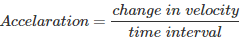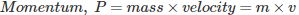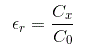# MCQs on Electrical And Electronics Measurements

##### Page 2 of 19. Go to page 1 2 3 4 5 6 7 8 9 10 11 12 13 14 15 16 17 18 19
01․ What are the SI units of mass, length and time respectively?
g, m and s.
kg, cm and s.
g, cm and s.
kg, m and s.

Kilogram, metre and second are the SI units of mass, length and time respectively.

02․ Which of the two have same dimensions?
Force and strain
Angular velocity and angular frequency
Force and stress
None of the above

Angular Velocity (ω) is change in angular displacement per unit time. It is also expressed as linear velocity/displacement. So, dimension of ‘ω’= MoLT -1/L = T -1. Angular Frequency (f) is defined as rate of change of angular displacement. It is the reciprocal of time period. So dimension of ‘f’ is T -1. Hence, dimensional formula of angular velocity is same as dimensional formula of angular frequency.

03․ What is the expression for acceleration?
Mass x velocity
Change in velocity / time
Mass x acceleration
Force x displacement04․ How many fundamental units are there ?
4
6
8
7

There are seven fundamental units: kilogram (mass), meter(length), candela (luminous intensity), second (time), ampere (current), kelvin (temperature), and mole (amount of substance).

05․ What is the expression for momentum?
force × displacement
mass × velocity
mass × acceleration
change of velocity / time06․ What is the unit of plane angle?
Candela
Degree

07․ Resistance of a VARISTOR varies with
Current
Voltage
Temperature
Both Voltage and Temperature

A varistor (variable resistor) is an electronic component whose electronic resistance varies with the applied voltage. It is also known as voltage dependent resistor (VDR).

08․ Relative permittivity can be measured by _______ bridge
Wheatstones.
Hays.
Desautys.
Schering.

The word relative permittivity is associated with the capacitance. Since, the Scherings bridge is used to find out the unknown value of capacitance, dielectric loss, relative permittivity. After finding the capacitance, we can find out the relative permittivity by using the formula,Here, C0 is the capacitance of a test capacitor and the Cx is the measured capacitance by Scherings bridge.

09․ _______ is used to measure the flow of air around aeroplane.
Anemometer.
Venturimeter.
Orifice.
nucleus.

The basic application of anemometer is to measure the wind velocity (or) flow of air. It is applicable in aeroplane, wind mills, etc.

10․ Null type recorders are
bridge recorders.
LVDT recorders.
potentiometric recorders.
any one of the above.

The recorders based on the working principle of self balancing or null conditions are called potentiometric recorders.

<<<1234>>>